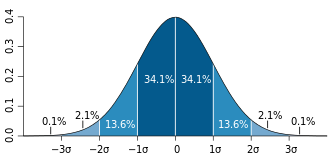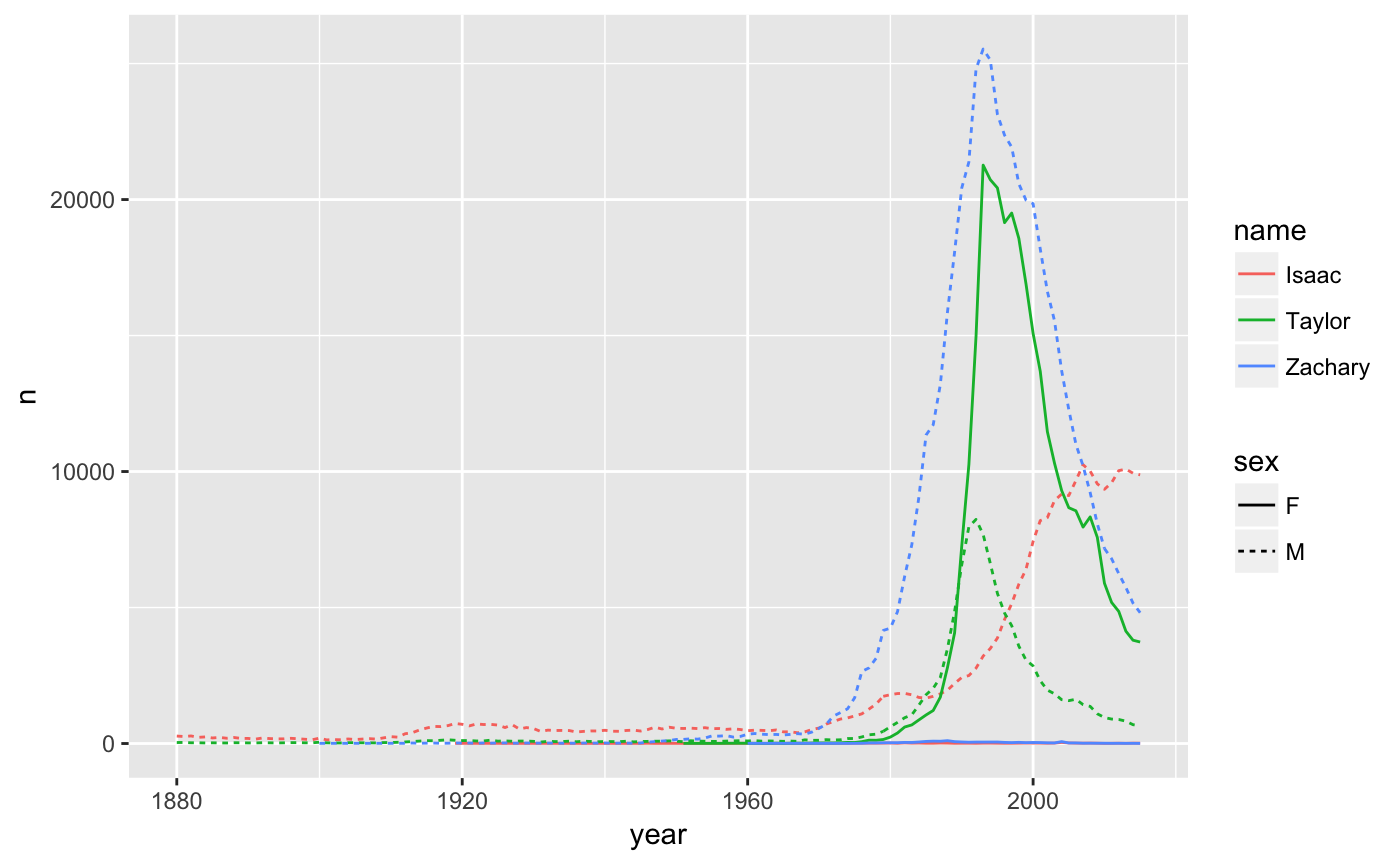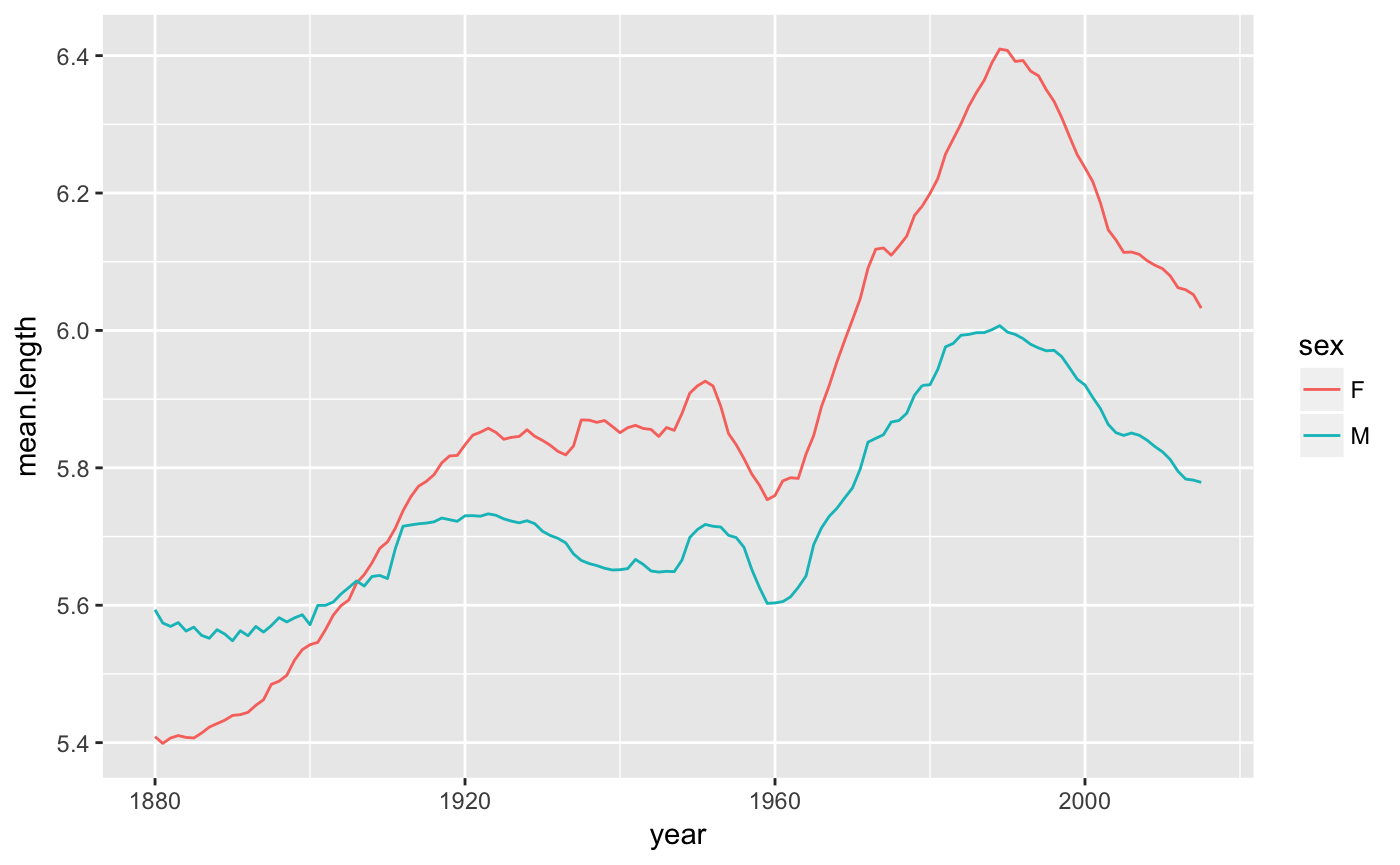Plan:

Revision Consolodate visualisation skills by going over homework solutions

New Explore ways to summarise numerical data

# Some simple statistics

• Characterising a sample
• range
• mean
• median
• Variance and standard deviation
• sd
• Distributions and histograms
• rnorm
• runif

### Revision: some less familiar operators

Integer division: %/%

• This gives you the whole number part of a division
7 %/% 2
 3

You can do this with a series of numerals

1:10 %/% 3
  0 0 1 1 1 2 2 2 3 3

Modulo %%:

• This gives you the remainder after you’ve done division.
17 %/% 3
 5
17 %% 3
 2

This is often used to tell if a number is even or odd:

#numbers <- 1:10
numbers <- c(4,45,2,46,96,75,32)
numbers %% 2
 0 1 0 0 0 1 0
numbers %% 2 == 1
 FALSE  TRUE FALSE FALSE FALSE  TRUE FALSE

Selecting the odd numbers

numbers[numbers %% 2 == 1]
 45 75

Selecting the even numbers

numbers[numbers %% 2 == 0]
  4  2 46 96 32

NOTE that this only works for integers (whole numbers); R often works with numeric values, which can also be decimals.

• cf. the is.integer() and as.integer() functions

## Mean

We’ll look at this again later, but here’s a reminder about the runif() function to generate random numbers

runif(10) # by default from 0 to 1
  0.3561 0.8718 0.1580 0.7354 0.9886 0.6004 0.7171 0.9770 0.4326 0.7649
runif(10, 0, 100) # or specify min max
  82.735  8.145 84.839  8.281  8.890  9.847 54.866 13.696 51.634 77.782
as.integer(runif(10,0,100))
   1 42 62 31 35 84 84 52 90 31
• Make a function to calculate the mean
• needs sum and length
values <- runif(30, 17, 987)
sum(values) / length(values)
 490.3
# my_func <- function(arg1) {do something with arg1}
custom.mean.func <- function(v) { sum(v)/length(v)}
custom.mean.func(values)
 490.3
custom.mean.func(1:10)
 5.5
mean(values) ## the built-in function
 490.3
• Although there is actually a mean() function that does exactly what you’d expect
• Handling NAs
values <- NA
values
  295.28  21.33     NA 243.24 720.07 963.24 494.86 432.98 841.42 801.84 946.57 748.44  92.99 392.27 960.72 423.76 409.10 299.33 839.03  48.92 282.76
 128.44 315.35 496.87 773.54 101.12 652.96 193.01 492.19 412.95
mean(values)
 NA
mean(values, na.rm=TRUE)
 476.7
custom.mean.func <- function(v, na.rm=FALSE) {
sum(v, na.rm=na.rm)/length(v[!is.na(v)])}
custom.mean.func(values, na.rm=TRUE)
 476.7

## Median

The middle value of a sorted series; if there are an even number of values then take the value halfway between the middle two.

values <- runif(21, 1, 55)
mean(values)
 26.62
median(values)
 23.72
# sort the values
sort(values)
   2.002  4.365  7.692 11.380 13.674 15.092 16.171 17.316 21.775 21.977 23.724 25.052 27.305 31.939 36.334 41.505 43.295 44.519 45.651 53.712 54.597
# the index for the midpoint is integer-half plus one
(length(values) %/% 2) + 1
 11
# use this number to index the sorted vector
sort(values)
 23.72
# put it all together
sort(values)[(length(values) %/% 2) + 1]
 23.72
odd.median <- function(v) {sort(v)[(length(v) %/% 2) + 1]}
# some tests
values <- runif(101, 0, 10)
odd.median(values)
 4.927
median(values)
 4.927
values <- runif(100, 0, 10)
odd.median(values)
 5.355
median(values)
 5.3
even.median <- function(v){
v.1 = sort(v)[length(v) %/% 2]
v.2 = sort(v)[(length(v) %/% 2) + 1]
(v.1 + v.2) / 2
}
even.median(values)
 5.3
median(values)
 5.3
universal.median <- function(v){
if (length(v) %% 2 == 1) { ## ODD
sort(v)[(length(v) %/% 2) + 1]
} else { ## EVEN
v.1 = sort(v)[length(v) %/% 2]
v.2 = sort(v)[(length(v) %/% 2) + 1]
(v.1 + v.2) / 2
}
}

Let’s run some tests

library(testthat)
for (i in 10:20){
values <- runif(i, 0, 100)
#print(universal.median(values) == median(values))
expect_equal(universal.median(values), median(values))
}
• Make your own function to calculate the median
• sort function
• %% and %/% (to carry out calculations over the indexes of the vector)
• Compare the output of your function to the built-in median function using the testthat package
• When is mean useful and when is median useful
income <- runif(100, 1000, 1500)
mean(income)
 1264
median(income)
 1263

But now someone really wealthy joins

income.2 <- c(income, 1000000)
mean(income.2)
 11153
median(income.2)
 1264

## Range

Knowing the range of a set of numbers can be useful

• min
• max
• range

Test these all out

values <- runif(10, 0, 100)
min(values)
 8.117
max(values)
 87.95
range(values)
  8.117 87.952

Problem: doesn’t handle outliers well Solution: look at the variance

## Population variance

The following shows how much each of these values differs from the mean value

values
  58.553 66.401 53.200 78.226 39.967 49.443 10.270 87.952  8.117 63.363
values - mean(values)
    7.004  14.851   1.651  26.677 -11.583  -2.106 -41.279  36.402 -43.432  11.814

Problem if we want the average difference from the mean, since some are positive and some are negative:

sum(values - mean(values))
 0

Solution is to square the values (in addition this has the generally desirable side effect of emphasising the larger values over the smaller values)

values - mean(values)
    7.004  14.851   1.651  26.677 -11.583  -2.106 -41.279  36.402 -43.432  11.814
(values - mean(values))^2
    49.056  220.567    2.726  711.661  134.156    4.437 1703.935 1325.135 1886.363  139.574
# The average square of the difference from the mean
sum((values - mean(values))^2) / length(a.c)
Error: object 'a.c' not found

The average square of the difference from the mean is called the population variance

This assumes that we are working with population, not a sample. The population is everything, the sample is a random subset.

Summary

• $$\mu$$ is the population mean (can be difficult or impossible to know)
• We could look at how much each value differs from the mean:

The average of this would be a nice statement of how far off the mean the values tend to be: $\frac{\Sigma(x_i - \mu)}{N}$ But there’s a problem, because these add up to zero

i.e. zero (sometimes this will give an extremely small number instead of zero — the precision of a computer is less than your human precision since the computer has to convert all these values to binary)

The solution: square it $\sigma^2 = \frac{\Sigma(x_i - \mu)^2}{N}$

• Guaranteed positive values
• Big differences from the mean are more heavily weighted than small differences
• Note $$\sigma^2$$ is the symbol for population variance

## Sample variance

• var

What if we don’t know the true mean? If we have a sample of the members of the population rather than the whole population.

• We use the sample mean rather than true mean in the equation
• We replace N by N - 1 (mathematical proof is hard, but basicially: the sample mean is always closer to the middle of the sample than the true mean, so using the population variance formula on a sample underestimates the value of the variance. Dividing by N-1 rather than by N increases the estimate of the variance slightly to compensate for this)

$s^2 = \frac{\Sigma(x_i - \bar{x})^2}{N - 1}$

• The symbol for sample variance is $$s^2$$
• The r function var() can be used to calculate the sample variance

## Standard deviation (population and sample)

• sd

Variance is kind of abstract

The solution is simply to take the square root. This is called the standard deviation

Usually we use the sample standard deviation: $s = \sqrt{\frac{\Sigma(x_i - \bar{x})^2}{N - 1}}$

There is also a population standard deviation, for when you are working with all the values that exist: $\sigma = \sqrt{\frac{\Sigma(x_i - \mu)^2}{N}}$ - The r function sd() calculates the sample standard deviation (see help(sd)) - Just for practice, make your own function to calculate sample standard deviation and compare it to the built-in function (using testthat package) - Make a function to calculate population standard devation and compare the values to sample standard deviation

## Normal distribution

Galton Board

In a normal distribution: - 68.2% of values within one standard deviation - 95.4% within two standard deviations - 99.7% within 3 - 99.99% within 4Standard deviations

Normal distributions can be described by two parameters, mean and standard deviation. The rnorm() function generates vectors of random numbers accordingn to a normal distribution.

• Read the help for the rnorm() function. Generate some vectors of normally distributed random numbers given different means and standard deviations.
• Graph them and work out their means and standard deviations from the vectors

## Histogram

• rnorm(N, mean, sd)

Here’s how you can visualise these:

A small sample will be jagged, but the larger the sample the smoother it gets:

## Uniform distribution

There are many kinds of distributions.

• runif(N, min, max)

In the Uniform distribution there is an equal chance of getting any value between the minimum and the maximum

# HOMEWORK NOTES

library(babynames)
library(stringr)

Anna’s name plot compares four dimensions (year, popularity, name, sex)

babynames%>%
filter(name=="Taylor"|name=="Zachary"|name=="Isaac")%>%
ggplot(aes(x=year, y=n, colour=name, linetype=sex)) + geom_line()Plotting mean name length of boys and girls (adapted from Bror-Magnus)

babynames %>%
group_by(year, sex) %>%
summarise(mean.length=sum(n*nchar(name))/sum(n)) %>%
ggplot(aes(x=year, y=mean.length, colour=sex)) + geom_line()Maja’s investigation of Chantal variants

1. testing the regular expression:
babynames\$name %>% unique() %>% str_subset("[S|C+]c?+h?+a+n{1,}+t+[a{1,}e{1,}]+l{1,}+e?") 
  "Chantal"   "Chantel"   "Chantell"  "Chantelle" "Shantel"   "Chantele"  "Shantell"  "Shantelle" "Chantale"  "Chantella" "Shantele"  "Shantella"
 "Shantal"   "Shantale"  "Chantalle" "Schantel"  "Shantala"  "Chanteal"  "Chantall"  "Shantela"  "Shantall"  "Shanteal"  "Shantalle"
1. plotting:

Linnea’s Game of Thrones plot. Note the use of annotate to put the label onto an independent layer:

filter(babynames, name=="Daenerys" | name=="Cersei" | name=="Arya" | name=="Sansa" | name=="Margaery" | name=="Ygritte" | name=="Brienne" | name=="Robb" | name=="Theon" | name=="Sandor" | name=="Tormund" | name=="Gendry" | name=="Jorah" | name=="Joffrey")%>% filter(year%in%2000:2015) %>% ggplot(aes(x=year, y=log(n), colour=name, linetype=sex)) + geom_line() + annotate("text", hjust=0, label="GoT release", x=2011, y=5.2) + geom_vline(xintercept=2011)